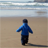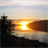# New to Qlik Sense

If you’re new to Qlik Sense, start with this Discussion Board and get up-to-speed quickly.

Announcements
Our May 2021 end-to-end product release from Data Integration to Data Analytics is out! READ DETAILS
cancel
Showing results for
Did you mean:Contributor III

## Nested IF Statements

Hello Qlik Users,

I need to have some nested if statements to turn ranges of scores into buckets.

My field is 'Total Score' and I need a bucket for scores greater than 99.99, scores between 99.99-95, and 94-90.

If some one could help with the syntax for multiple if statements that would be great.

Thanks

1 Solution

Accepted SolutionsNot applicable

Hi

If( [Total Score] > 99.99, 'A',

If([Total Score] <=99.99 and [Total Score] >=95),'B',

If([Total Score] <95 and [Total Score] >=90,'C','D')))

17 RepliesMVP

Maybe something like

If( [Total Score] > 99.99, dual( '> 99.99', 100),

If([Total Score] >=95, Dual('95 - 99.99', 95),

If([Total Score] >=90 , Dual('90 - 95', 90) )

)

)Champion

Did you check this, clean explanation of Bucket with multiple IF statement?

BucketsChampion

IF( Condition  , True, If( Condition , True) )  Hope this helps.

vikasNot applicable

hi  IF(material4='TAB','TAB',IF(material4='SMP','SMP','REL')) AS REL4, bjut its working fine i need to reflet this  material4 to REL4.Not applicable

Hi

If( [Total Score] > 99.99, 'A',

If([Total Score] <=99.99 and [Total Score] >=95),'B',

If([Total Score] <95 and [Total Score] >=90,'C','D')))Not applicable

Is there a more efficient method to nested if statements?

In Excel VBA I would use the "Case Select" function.  Is there something similar in Qlikview?Contributor II

If( [Total Score] > 99.99, 'A',

If([Total Score] <=99.99 and [Total Score] >=95),'B',

If([Total Score] <95 and [Total Score] >=90,'C','D'))Not applicable

How would I nest the IF statements using the following script?

IF([Expenditure Type]='FB, Classified Staff','FB',)

IF([Expenditure Type]='FB, Univ Staff,'FB',)

IF([Expenditure Type]=’Awards & Prizes (Cash)’,’Reward &  Recognition’,)

IF([Expenditure Type]= 'Emp, Recognition Awrd-Non Txbl','Reward  & Recognition',)

IF([Expenditure Type]= 'Employee, Recognition Awards','Reward  &  Recognition',)

IF([Expenditure Type]= ‘Eq Capital Network Servers’,'Hardware’,)Contributor II

you have to nested if statement for this expression

if statement contains comma (,) is represented by "else" part .

In your else part put after comma.Tags